# Osserman conjecture

(diff) ← Older revision | Latest revision (diff) | Newer revision → (diff)

Letbe the Riemann curvature tensor of a Riemannian manifold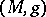. Let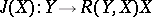be the Jacobi operator. Ifis a unit tangent vector at a pointof, then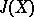is a self-adjoint endomorphism of the tangent bundle at. Ifis flat or is locally a rank-symmetric space (cf. also Symmetric space), then the set of local isometries acts transitively on the sphere bundleof unit tangent vectors, sohas constant eigenvalues on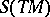. R. Osserman [a6] wondered if the converse implication was valid; the following conjecture has become known as the Osserman conjecture: Ifhas constant eigenvalues, thenis flat or is locally a rank-symmetric space.

Letbe the dimension of. Ifis odd, ifmodulo, or if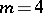, then C.S. Chi [a3] has established this conjecture using a blend of tools from algebraic topology and differential geometry. There is a corresponding purely algebraic problem. Letbe a-tensor on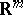which defines a corresponding curvature operator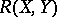. Ifsatisfies the identities,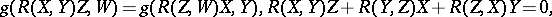thenis said to be an algebraic curvature tensor. The Riemann curvature tensor of a Riemannian metric is an algebraic curvature tensor. Conversely, given an algebraic curvature tensor at a pointof, there always exists a Riemannian metric whose curvature tensor atis. Let; this is a self-adjoint endomorphism of the tangent bundle at. One says thatis Osserman if the eigenvalues ofare constant on the unit sphere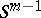in. C.S. Chi classified the Osserman algebraic curvature tensors forodd ormodulo; he then used the second Bianchi identity to complete the proof. However, if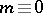modulo, it is known [a4] that there are Osserman algebraic curvature tensors which are not the curvature tensors of rank-symmetric spaces and the classification promises to be considerably more complicated in these dimensions.

There is a generalization of this conjecture to metrics of higher signature. In the Lorentzian setting, one can show that any algebraic curvature tensor which is Osserman is the algebraic curvature tensor of a metric of constant sectional curvature; it then follows that any Osserman Lorentzian metric has constant sectional curvature [a2]. For metrics of higher signature, the Jordan normal form of the Jacobi operator enters; the Jacobi operator need not be diagonalizable. There exist indefinite metrics which are not locally homogeneous, so thatis nilpotent for all tangent vectors, see, for example, [a5].

If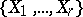is an orthonormal basis for an-plane, one can define a higher-order Jacobi operator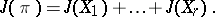One says that an algebraic curvature tensor or Riemannian metric is-Osserman if the eigenvalues ofare constant on the Grassmannian of non-oriented-planes in the tangent bundle. I. Stavrov [a8] and G. Stanilov and V. Videv [a7] have obtained some results in this setting.

In the Riemannian setting, ifI. Dotti, M. Druetta and P. Gilkey [a1] have recently classified the-Osserman algebraic curvature tensors and showed that the only-Osserman metrics are the metrics of constant sectional curvature.

How to Cite This Entry:
Osserman conjecture. Encyclopedia of Mathematics. URL: http://encyclopediaofmath.org/index.php?title=Osserman_conjecture&oldid=15029
This article was adapted from an original article by P.B. Gilkey (originator), which appeared in Encyclopedia of Mathematics - ISBN 1402006098. See original article